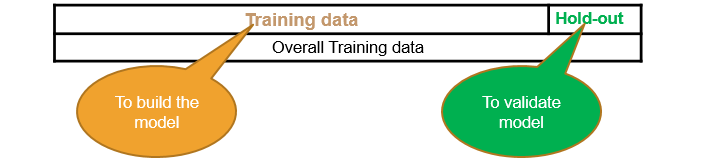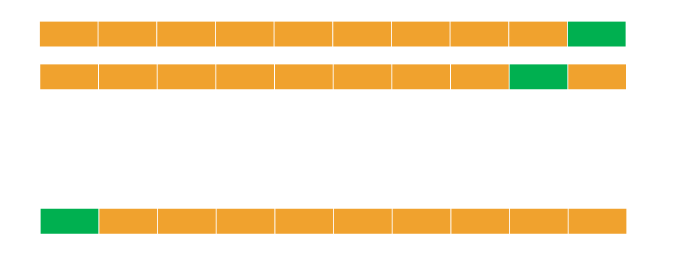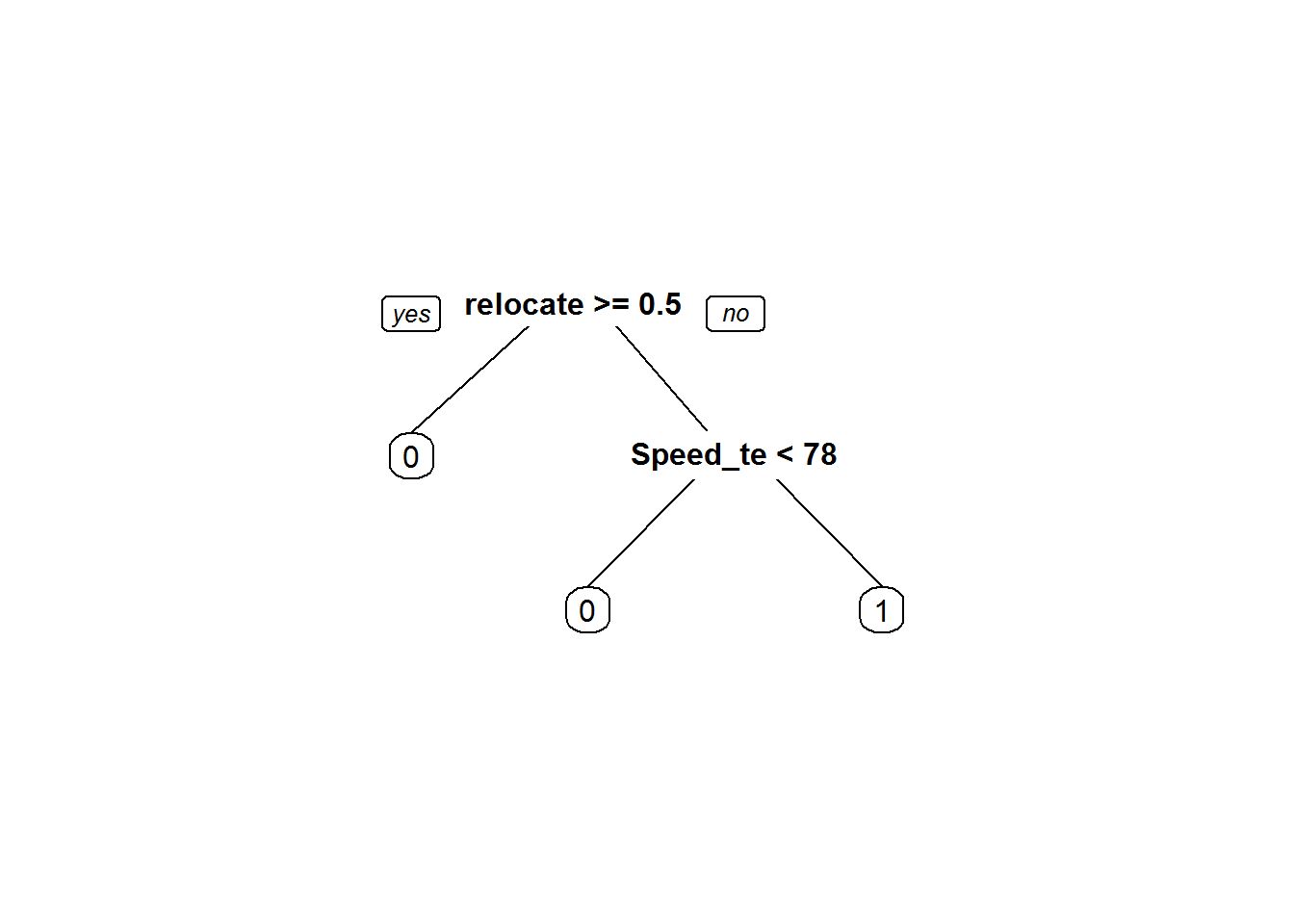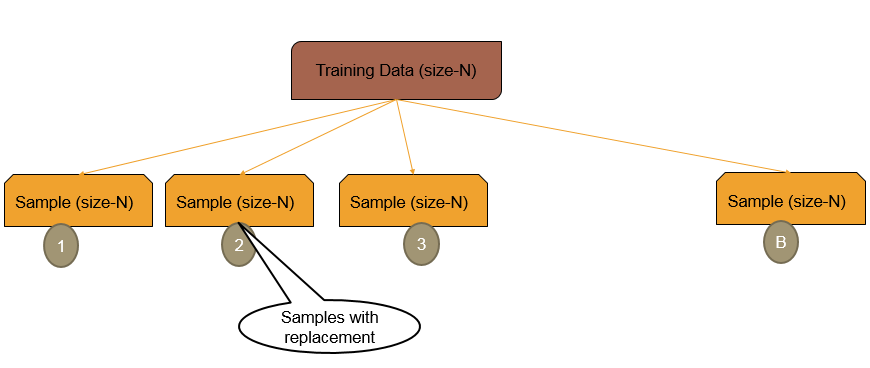• No products in the cart.

# 203.4.7 Cross Validation

### Choosing Optimal Model

• Unfortunately there is no scientific method of choosing optimal model complexity that gives minimum test error.
• Training error is not a good estimate of the test error.
• There is always bias-variance tradeoff in choosing the appropriate complexity of the model.
• We can use cross validation methods, boot strapping and bagging to choose the optimal and consistent model

### Holdout Data Cross Validation

• The best solution is out of time validation. Or the testing error should be given high priority over the training error.
• A model that is performing good on training data and equally good on testing is preferred.
• We may not have the test data always. How do we estimate test error?
• We take the part of the data as training and keep aside some potion for validation. May be 80%-20% or 90%-10%
• Data splitting is a very basic intuitive method### LAB: Holdout Data Cross Validation

• Data: Fiberbits/Fiberbits.csv
• Take a random sample with 80% data as training sample
• Use rest 20% as holdout sample.
• Build a model on 80% of the data. Try to validate it on holdout sample.
• Try to increase or reduce the complexity and choose the best model that performs well on training data as well as holdout data

### Solution

• Caret is a good package for cross validation
library(caret)
sampleseed <- createDataPartition(Fiberbits$active_cust, p=0.80, list=FALSE) train_new <- Fiberbits[sampleseed,] hold_out <- Fiberbits[-sampleseed,] • Model1 library(rpart) Fiber_bits_tree5<-rpart(active_cust~., method="class", control=rpart.control(minsplit=5, cp=0.000001), data=train_new) Fbits_pred5<-predict(Fiber_bits_tree5, type="class") • Accuracy on Training Data conf_matrix5<-table(Fbits_pred5,train_new$active_cust)
conf_matrix5
##
## Fbits_pred5     0     1
##           0 31482  1689
##           1  2230 44599
accuracy5<-(conf_matrix5[1,1]+conf_matrix5[2,2])/(sum(conf_matrix5))
accuracy5
##  0.9510125
• Model1 Validation accuracy
hold_out$pred <- predict(Fiber_bits_tree5, hold_out, type="class") conf_matrix_val<-table(hold_out$pred,hold_out$active_cust) conf_matrix_val ## ## 0 1 ## 0 7003 1333 ## 1 1426 10238 accuracy_val<-(conf_matrix_val[1,1]+conf_matrix_val[2,2])/(sum(conf_matrix_val)) accuracy_val ##  0.86205 • Model2 Fiber_bits_tree5<-rpart(active_cust~., method="class", control=rpart.control(minsplit=30, cp=0.05), data=train_new) Fbits_pred5<-predict(Fiber_bits_tree5, type="class") conf_matrix5<-table(Fbits_pred5,train_new$active_cust)
• Accuracy on Training Data
accuracy5<-(conf_matrix5[1,1]+conf_matrix5[2,2])/(sum(conf_matrix5))
accuracy5
##  0.7882375
• Model2 Validation accuracy
hold_out$pred <- predict(Fiber_bits_tree5, hold_out,type="class") conf_matrix_val<-table(hold_out$pred,hold_out$active_cust) accuracy_val<-(conf_matrix_val[1,1]+conf_matrix_val[2,2])/(sum(conf_matrix_val)) accuracy_val ##  0.79225 • Model3 Fiber_bits_tree5<-rpart(active_cust~., method="class", control=rpart.control(minsplit=30, cp=0.001), data=train_new) Fbits_pred5<-predict(Fiber_bits_tree5, type="class") conf_matrix5<-table(Fbits_pred5,train_new$active_cust)
• Accuracy on Training Data
accuracy5<-(conf_matrix5[1,1]+conf_matrix5[2,2])/(sum(conf_matrix5))
accuracy5
##  0.8673
• Model3 Validation accuracy
hold_out$pred <- predict(Fiber_bits_tree5, hold_out,type="class") conf_matrix_val<-table(hold_out$pred,hold_out$active_cust) accuracy_val<-(conf_matrix_val[1,1]+conf_matrix_val[2,2])/(sum(conf_matrix_val)) accuracy_val ##  0.8661 ### Ten-fold Cross – Validation • Divide the data into 10 parts(randomly) • Use 9 parts as training data(90%) and the tenth part as holdout data(10%) • We can repeat this process 10 times • Build 10 models, find average error on 10 holdout samples. This gives us an idea on testing error### K-fold Cross Validation • A generalization of cross validation. • Divide the whole dataset into k equal parts • Use kth part of the data as the holdout sample, use remaining k-1 parts of the data as training data • Repeat this K times, build K models. The average error on holdout sample gives us an idea on the testing error • Which model to choose? • Choose the model with least error and least complexity • Or the model with less than average error and simple (less parameters) • Finally use complete data and build a model with the chosen number of parameters • Note: Its better to choose K between 5 to 10. Which gives 80% to 90% training data and rest 20% to 10% is holdout data ### LAB – K-fold Cross Validation • Build a tree model on the fiber bits data. • Try to build the best model by making all the possible adjustments to the parameters. • What is the accuracy of the above model? • Perform 10 -fold cross validation. What is the final accuracy? • Perform 20 -fold cross validation. What is the final accuracy? • What can be the expected accuracy on the unknown dataset? ### Solution • Model on complete training data Fiber_bits_tree3<-rpart(active_cust~., method="class", control=rpart.control(minsplit=10, cp=0.000001), data=Fiberbits) Fbits_pred3<-predict(Fiber_bits_tree3, type="class") conf_matrix3<-table(Fbits_pred3,Fiberbits$active_cust)
conf_matrix3
##
## Fbits_pred3     0     1
##           0 38154  2849
##           1  3987 55010
• Accuracy on Traing Data
accuracy3<-(conf_matrix3[1,1]+conf_matrix3[2,2])/(sum(conf_matrix3))
accuracy3
##  0.93164
• k-fold Cross Validation building
• K=10
library(caret)
train_dat <- trainControl(method="cv", number=10)

Need to convert the dependent variable to factor before fitting the model

Fiberbits$active_cust<-as.factor(Fiberbits$active_cust)
• Building the models on K-fold samples
library(e1071)
## Warning: package 'e1071' was built under R version 3.1.3
K_fold_tree<-train(active_cust~., method="rpart", trControl=train_dat, control=rpart.control(minsplit=10, cp=0.000001),  data=Fiberbits)
K_fold_tree$finalModel ## n= 100000 ## ## node), split, n, loss, yval, (yprob) ## * denotes terminal node ## ## 1) root 100000 42141 1 (0.42141000 0.57859000) ## 2) relocated>=0.5 12348 954 0 (0.92274052 0.07725948) * ## 3) relocated< 0.5 87652 30747 1 (0.35078492 0.64921508) ## 6) Speed_test_result< 78.5 27517 10303 0 (0.62557692 0.37442308) * ## 7) Speed_test_result>=78.5 60135 13533 1 (0.22504365 0.77495635) * prp(K_fold_tree$finalModel)Kfold_pred<-predict(K_fold_tree)
conf_matrix6<-confusionMatrix(Kfold_pred,Fiberbits$active_cust) conf_matrix6 ## Confusion Matrix and Statistics ## ## Reference ## Prediction 0 1 ## 0 28608 11257 ## 1 13533 46602 ## ## Accuracy : 0.7521 ## 95% CI : (0.7494, 0.7548) ## No Information Rate : 0.5786 ## P-Value [Acc > NIR] : < 2.2e-16 ## ## Kappa : 0.4879 ## Mcnemar's Test P-Value : < 2.2e-16 ## ## Sensitivity : 0.6789 ## Specificity : 0.8054 ## Pos Pred Value : 0.7176 ## Neg Pred Value : 0.7750 ## Prevalence : 0.4214 ## Detection Rate : 0.2861 ## Detection Prevalence : 0.3987 ## Balanced Accuracy : 0.7422 ## ## 'Positive' Class : 0 ##  • K=20 library(caret) train_dat <- trainControl(method="cv", number=20) Need to convert the dependent variable to factor before fitting the model Fiberbits$active_cust<-as.factor(Fiberbits$active_cust) Building the models on K-fold samples library(e1071) K_fold_tree_1<-train(active_cust~., method="rpart", trControl=train_dat, control=rpart.control(minsplit=10, cp=0.000001), data=Fiberbits) K_fold_tree_1$finalModel
## n= 100000
##
## node), split, n, loss, yval, (yprob)
##       * denotes terminal node
##
## 1) root 100000 42141 1 (0.42141000 0.57859000)
##   2) relocated>=0.5 12348   954 0 (0.92274052 0.07725948) *
##   3) relocated< 0.5 87652 30747 1 (0.35078492 0.64921508)
##     6) Speed_test_result< 78.5 27517 10303 0 (0.62557692 0.37442308) *
##     7) Speed_test_result>=78.5 60135 13533 1 (0.22504365 0.77495635) *
prp(K_fold_tree_1$finalModel)Kfold_pred<-predict(K_fold_tree_1) Caret package has confusion matrix function conf_matrix6_1<-confusionMatrix(Kfold_pred,Fiberbits$active_cust)
conf_matrix6_1
## Confusion Matrix and Statistics
##
##           Reference
## Prediction     0     1
##          0 28608 11257
##          1 13533 46602
##
##                Accuracy : 0.7521
##                  95% CI : (0.7494, 0.7548)
##     No Information Rate : 0.5786
##     P-Value [Acc > NIR] : < 2.2e-16
##
##                   Kappa : 0.4879
##  Mcnemar's Test P-Value : < 2.2e-16
##
##             Sensitivity : 0.6789
##             Specificity : 0.8054
##          Pos Pred Value : 0.7176
##          Neg Pred Value : 0.7750
##              Prevalence : 0.4214
##          Detection Rate : 0.2861
##    Detection Prevalence : 0.3987
##       Balanced Accuracy : 0.7422
##
##        'Positive' Class : 0
## 

## Bootstrap Cross Validation

### Bootstrap Methods

• Boot strapping is a powerful tool to get an idea on accuracy of the model and the test error
• Can estimate the likely future performance of a given modeling procedure, on new data not yet realized.
• The Algorithm
• We have a training data is of size N
• Draw random sample with replacement of size N – This gives a new dataset, it might have repeated observations, some observations might not have even appeared once.
• Create B such new datasets. These are called boot strap datasets
• Build the model on these B datasets, we can test the models on the original training dataset.### Bootstrap Example

• Example
1. We have a training data is of size 500
2. Boot Strap Data-1:
• Create a dataset of size 500. To create this dataset, draw a random point, note it down, then replace it back. Again draw another sample point. Repeat this process 500 times. This makes a dataset of size 500. Call this as Boot Strap Data-1
1. Multiple Boot Strap datasets
• Repeat the procedure in step -2 multiple times. Say 200 times. Then we have 200 Boot Strap datasets
1. We can build the models on these 200 boost strap datasets and the average error gives a good idea on overall error. We can even use the original training data as the test data for each of the models

### LAB: Bootstrap Cross Validation

• Draw a boot strap sample with sufficient sample size
• Build a tree model and get an estimate on true accuracy of the model

### Solution

• Draw a boot strap sample with sufficient sample size

Where number is B

train_control <- trainControl(method="boot", number=20) 

Tree model on boots straped data

Boot_Strap_model <- train(active_cust~., method="rpart", trControl= train_control, control=rpart.control(minsplit=10, cp=0.000001),  data=Fiberbits)
Boot_Strap_predictions <- predict(Boot_Strap_model)

conf_matrix7<-confusionMatrix(Boot_Strap_predictions,Fiberbits\$active_cust)
conf_matrix7
## Confusion Matrix and Statistics
##
##           Reference
## Prediction     0     1
##          0 28608 11257
##          1 13533 46602
##
##                Accuracy : 0.7521
##                  95% CI : (0.7494, 0.7548)
##     No Information Rate : 0.5786
##     P-Value [Acc > NIR] : < 2.2e-16
##
##                   Kappa : 0.4879
##  Mcnemar's Test P-Value : < 2.2e-16
##
##             Sensitivity : 0.6789
##             Specificity : 0.8054
##          Pos Pred Value : 0.7176
##          Neg Pred Value : 0.7750
##              Prevalence : 0.4214
##          Detection Rate : 0.2861
##    Detection Prevalence : 0.3987
##       Balanced Accuracy : 0.7422
##
##        'Positive' Class : 0
## 

## Conclusion

• We studied
• Validating a model, Types of data & Types of errors
• The problem of over fitting & The problem of under fitting
• Cross validation & Boot strapping
• Training error is what we see and that is not the true performance metric
• Test error plays vital role in model selection
• R-square, Adj-R-square, Accuracy, ROC, AUC, AIC and BIC can be used to get an idea on training error
• Cross Validation and Boot strapping techniques give us an idea on test error
• Choose the model based on the combination of AIC, Cross Validation and Boot strapping results
• Bootstrap is widely used in ensemble models & random forests.

In next section, we will be studying about Neural Networks

### 0 responses on "203.4.7 Cross Validation"

Statinfer Software Solutions LLP

Software Technology Parks of India,
NH16, Krishna Nagar, Benz Circle,